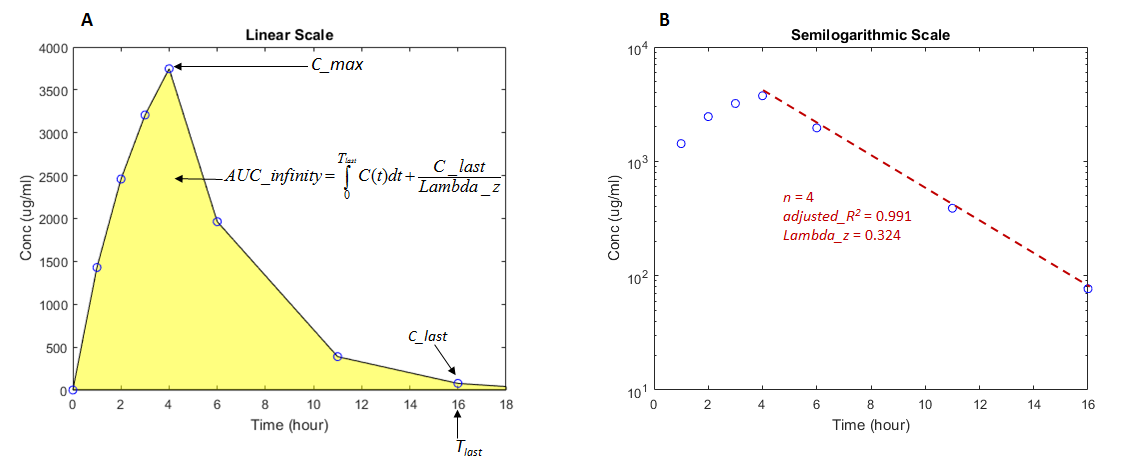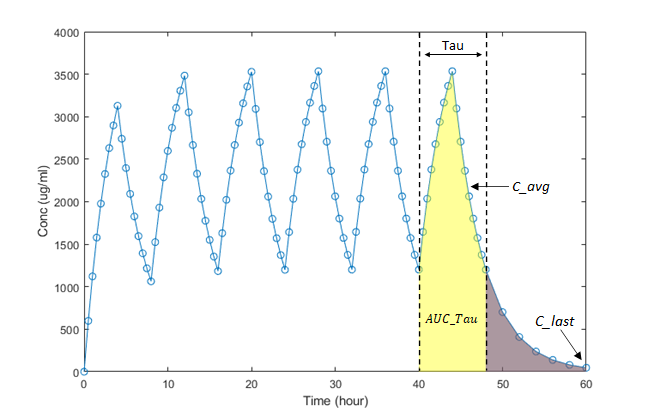Documentation

### This is machine translation

Mouseover text to see original. Click the button below to return to the English version of the page.

To view all translated materials including this page, select Country from the country navigator on the bottom of this page.

## Noncompartmental Analysis

Noncompartmental analysis (NCA) lets you compute pharmacokinetic (PK) parameters of a drug from the time course of measured drug concentrations. This approach does not require the assumption of a specific compartmental model. NCA is often used to determine the degree of exposure following administration of a drug, such as AUC, and other PK parameters, such as the clearance and the terminal half-life.

### Data

SimBiology® lets you calculate NCA parameters from concentration–time data. The data must contain a time column, a concentration column, and a dose column that defines dose amounts. Three types of drug administration routes are supported: IV bolus, IV infusion, and Extravascular. You can have a column for each type. For infusion doses, an infusion rate column is also needed.

If you have data containing multiple groups of observations, you can define a group column. If needed, you can use two levels of hierarchy to specify grouping. Specify the outer level of grouping using the group column, and specify the inner level (subgroups) in the ID column. Consider data that contains three groups, where each group contains four patients. The group column labels the three groups, and the ID column labels each patient.

### Dosing

Single-dosing data contains a single dose amount for each individual. Multiple-dosing data has several doses at different times for each individual. There are common parameters calculated for either type of dosing data, and parameters that are specific to single or multiple dosing.

#### Common Parameters for Single and Multiple Dosing

SimBiology computes some common parameters for single- or multiple-dosing data. This figure represents the concentration-time profile after a single dose. For multiple dosing, the same principles apply, except that SimBiology uses the first period, that is, after the first dose and before the second.Figure A shows concentration–time data in a linear scale and illustrates how the AUC from time 0 to infinity is calculated. Figure B shows the same data in a semilogarithmic scale. To compute the terminal rate constant (Lambda_z), SimBiology performs a set of linear regressions of the log-transformed data using each of the last n points (n = ```3, 4, 5, ...```) from the terminal portion of the curve. Lambda_z is chosen from the regression that uses the most points and has the maximum adjusted_R2.

This table describes the common parameters for single and multiple dosing.

ParameterDescription
AUC_0_last

Area under the measured concentration–time curve from time = 0 to the last time point.

$AUC_0_last=\underset{0}{\overset{Tlast}{\int }}C\left(t\right)dt$,

where C(t) is the plasma concentration at time t.

SimBiology uses the linear trapezoidal method (MATLAB) to calculate the AUC.

AUC_infinity

Total area under the concentration–time curve extrapolating to Inf using the terminal rate constant Lambda_z.

$AUC_infinity=AUC_0_last+\frac{C_last}{Lambda_z}$,

where C_last is the last observed concentration and Lambda_z is the terminal rate constant.

AUC_infinity_dose

$AUC_infinity_dose=\frac{AUC_infinity}{DM}$.

AUCx_yPartial AUC computed for a custom time range, where x and y are the start and end times, respectively.
AUC_extrap_percent

Fraction of total AUC_infinity obtained from extrapolation.

$AUC_extrap_percent=\frac{AUC_infinity-AUC_0_last}{AUC_infinity}*100$.

Lambda_z

To calculate the terminal rate constant (Lambda_z), SimBiology performs a set of linear regressions of the log(concentration)–time data using each of the last n points (```n = 3, 4, 5, ...```) from the terminal portion of the curve, that is, points satisfying the conditions: $\left(Time\ge {T}_{\mathrm{max}}\right)&\left(Conc\le {C}_{\mathrm{max}}\right)$. A minimum of three points is required to determine Lambda_z.

Lambda_z is chosen from the regression that uses the most points and has the maximum adjusted_R2 among all regressions.

$adjusted_{R}^{2}=1-\frac{\left(1-{R}^{2}\right)*\left(n-1\right)}{n-2}$

R2Coefficient of determination for the `linear regressions` used in the Lambda_z calculation.
Num_pointsNumber of data points from the profile used in the determination of Lambda_z.
CL

Total drug clearance.

$Cl=\frac{DM}{AUC_infinity}$,

where DM is the dose amount.

C_0

Extrapolated concentration at time = 0, computed using a regression of the first two data points in a profile. This parameter is for IV Bolus doses only.

C_max

Maximum observed concentration.

C_max_Dose

$C_\mathrm{max}_Dose=\frac{C_max}{DM}$.

C_max_x_yMaximum observed concentration within a given time range, specified by the start time x and the end time y. This parameter is computed when you specify a custom time range in the Cmax Time Range box in the SimBiology desktop or set the `C_max_ranges` property of the options object created by `sbioncaoptions`.
MRT

Mean residence time.

$MRT=\frac{AUMC}{AUC_infinity}$.

TlastTime of the last observed concentration value above the lower limit of quantization (LOQ).
T_half

Terminal half-life of the drug.

$T_half=\frac{\mathrm{ln}\left(2\right)}{Lambda_z}$.

T_max

T_max is the time point at which the maximum concentration (C_max) is observed.

T_max_x_yTime point at which maximum concentration is observed within a given time range, specified by the start time x and the end time y. This parameter is computed when you specify a custom time range in the Cmax Time Range box in the SimBiology desktop or set the `C_max_ranges` property of the options object created by `sbioncaoptions`.
V_ss

Apparent volume of distribution at equilibrium. This parameter is for IV Bolus doses only.

$V_ss=\frac{DM*AUMC}{AUC_infinit{y}^{2}}$.

V_z

Volume of distribution during the terminal phase.

$V_z=\frac{DM}{AUC_infinity*Lambda_z}$.

DM

Administered dose amount. For multiple dosing, the last administered dose is reported.

doseScheduleSingle- or multiple-dosing data.
administrationRouteDose administration route. Supported routes are `IVBolus`, `IVInfusion`, `ExtraVascular`.

#### Parameters for Single Dosing

In addition to the common parameters, SimBiology reports parameters for single-dosing data.

ParameterDescription
AUMC_0_last

Area under the first moment of the concentration–time curve from time 0 to the last time point Tlast.

$AUMC_0_last=\underset{0}{\overset{Tlast}{\int }}t*C\left(t\right)dt$.

AUMC

Total area under the first moment of the concentration–time curve extrapolating to Inf using Lambda_z.

$AUMC=AUMC_0_last+\frac{C_last}{Lambda_{z}^{2}}+\frac{Tlast*C_last}{Lambda_z}$.

AUMC_extrap_percent

Fraction of total AUMC obtained from extrapolation.

$AUMC_extrap_percent=\frac{AUMC-AUMC_0_last}{AUMC}*100$.

#### Parameters for Multiple Dosing

This figure shows the concentration-time profile after multiple doses. SimBiology uses the first period, that is, after the first dose and before the second, to compute the following NCA parameters for multiple-dosing data, in addition to the common parameters listed previously.ParameterDescription
AUC_Tau

Area under the concentration–time curve during a dosing period of length Tau. SimBiology uses the first period, that is, after the first dose and before the second.

$AUC_Tau={\int }_{T_first_period}^{T_first_period+Tau}C\left(t\right)dt$.

TauDosing interval.
C_Avg

Average concentration over one period.

$C_Avg=\frac{AUC_Tau}{Tau}$.

C_min

Minimum observed concentration during the first period, that is, ```C_min = C(T_min)```.

PTF_percent

Peak trough fluctuation over one dosing interval at steady state.

$PTF_Percent=\frac{C_\mathrm{max}-C_\mathrm{min}}{C_Avg}*100$.

Accumulation_Index

Theoretical accumulation ratio.

$Accumulation_Index=\frac{1}{1-{e}^{-Lambda_z*Tau}}$.

T_min

Time at which the minimum concentration is reached in a dosing interval.

Sparse Sampling.  To calculate PK parameters, measured concentrations at multiple time points for each individual is needed after the drug administration. Under certain circumstances, it is not feasible or not practical to obtain such longitudinal data on a single subject. In such cases, concentration data is collected from multiple individuals at each time point and then averaged to calculate NCA parameters for each group instead. SimBiology performs such sparse sampling by taking the average of the dependent variable for all individuals at the same time point. It then returns the values of NCA parameters for each group. Time values for each measurement across individuals (IDs) within a group must be identical.

### Calculating NCA Parameters

You can calculate NCA parameters using the `sbionca` function in the command line or using the SimBiology desktop.

#### Using sbionca

`sbionca` provides command line functionality to compute NCA parameters. Define the data classification options and parameter calculation options using an option object created by `sbioncaoptions`. For an example, see Compute NCA Parameters from Concentration-Time Data.

#### Using SimBiology Desktop

After you import the data, select Open > NCA on the Define Plot tab. If your data has a grouping column, specify it using Group Data Column. Use ID Data Column to specify the inner level of grouping. For details, see Data. Specify the dosing data column (IV Bolus Dose Data Column or Extravascular Dose Data Column, or both if you have two columns for both types). Lower limit of quantization (LOQ) is a threshold below which the values of dependent variables are truncated to zero.

Lambda Time Range lets you specify a custom time range to compute the terminal rate constant (Lambda_z). The time range applies to all groups; you cannot specify a different time range for each group.

Partial AUC lets you specify a custom time range to compute the partial AUC bounded by the start and end times. You can specify a different time range for each group.

Cmax Time Range lets you specify a custom time range to report the maximum observed concentration within the range (C_max) and the time (T_max) when it is observed. You can specify a different time range for each group.

To export the calculated statistics, select Export Statistics from the context menu of the NCA table. By default, the data is exported as a table (MATLAB). To convert it to a `dataset`, use `table2dataset`.